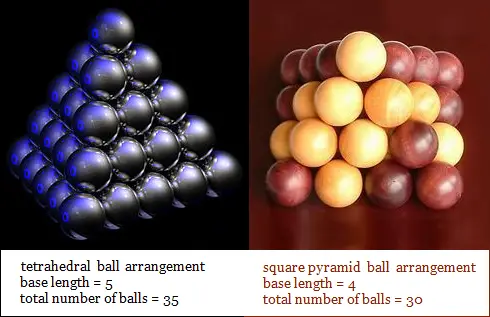# Pyramid of Balls Calculator

There are two ways to stack spheres into a pyramid. One is to start with a square base, adding layers of smaller squares on top until you reach the apex. Another is to start with a triangular base and build up the layers with successively smaller triangles. (See image below.)

Mathematically, both of these arrangements are the most efficient way to pack spheres in three dimensional space; each arrangement has a packing density of π/√18 = 0.74048. This means in either pyramidal formation the spheres occupy 74.048% of the available space.

The calculator below computes the dimensions of a pyramid of stacked spheres. Just input the type of arrangement, the number of balls along the edge of the base, and the diameter of each sphere.

 Pyramid Type:   Tetrahedron (Triangle Base)   Square Base Pyramid   Rectangle Base Pyramid Base Length in Balls = Base Width in Balls  = (Rectangle Only) Ball Diameter = inchescm### Balls Stacked in a Tetrahedron

When spheres of equal size are stacked into a pyramid with a triangular base, i.e., a tetrahedron, the number of balls on the bottom layer is n(n+1)/2, where n is the number of balls in the longest row along the base. The total number of balls in the pyramid is n(n+1)(n+2)/6.

The height of such a pyramid is a function of n and d, the diameter of each sphere. To find the height in terms of n and d, first imagine a tetrahedron made from four balls--three balls on the bottom and one ball at the top. The centers of these spheres form a tetrahedron with an edge length of d and a height of d2/3. The total height of stack of spheres is d + d2/3.

Now add a third layer to the bottom of the pyramid (add six balls for a total of 10 balls), and consider the tetrahedron formed by the centers of the spheres at the corners of the pyramid. This imaginary tetrahedron has an edge length of 2d and a height of 2d2/3. The total height of the stack is d + 2d2/3.

In general, the height is d + (n-1)d√2/3.

The base of the stack can be inscribed in an equilateral triangle with a side length of d(n-1) + d√3.

### Balls Stacked in a Square Pyramid

When spheres are stacked into a square pyramidal formation, the base layer contains n² balls, where n is the number of balls that form the side of the square. The total number of spheres in the pyramid is n(n+1)(2n+1)/6.

To find the height of the pyramidal stack in terms of n and d, first imagine a square pyramid made from five balls--four balls on the bottom and one ball at the top. The centers of these spheres form a square pyramid with an edge length of d and a height of d1/2. The total height of stack of spheres is d + d1/2. Each time you add a layer of balls to the bottom of the pyramid, the height increases by d1/2. Thus, for a square pyramidal formation with an edge length of n balls and a ball diameter of d, the height is d + d(n-1)√1/2.

The square that contains the base has a length of dn.

### Balls Stacked in a Rectangular Pyramid

If you stack spheres into a pyramid with a (non-square) rectangular base, the top of the pyramid will not consist of a single sphere but a line of spheres. If the shorter dimension of the base contains n balls and the longer dimension contains m balls, then the number of balls at the base is mn. The number of balls at the top is m - n + 1. The total number of balls is n(n+1)(3m-n+1)/6.

As in the square case, if each sphere has a diameter of d, the height of such a pyramid will be d + d(n-1)√1/2.

The dimensions of the rectangle that contains the base is dn by dm.